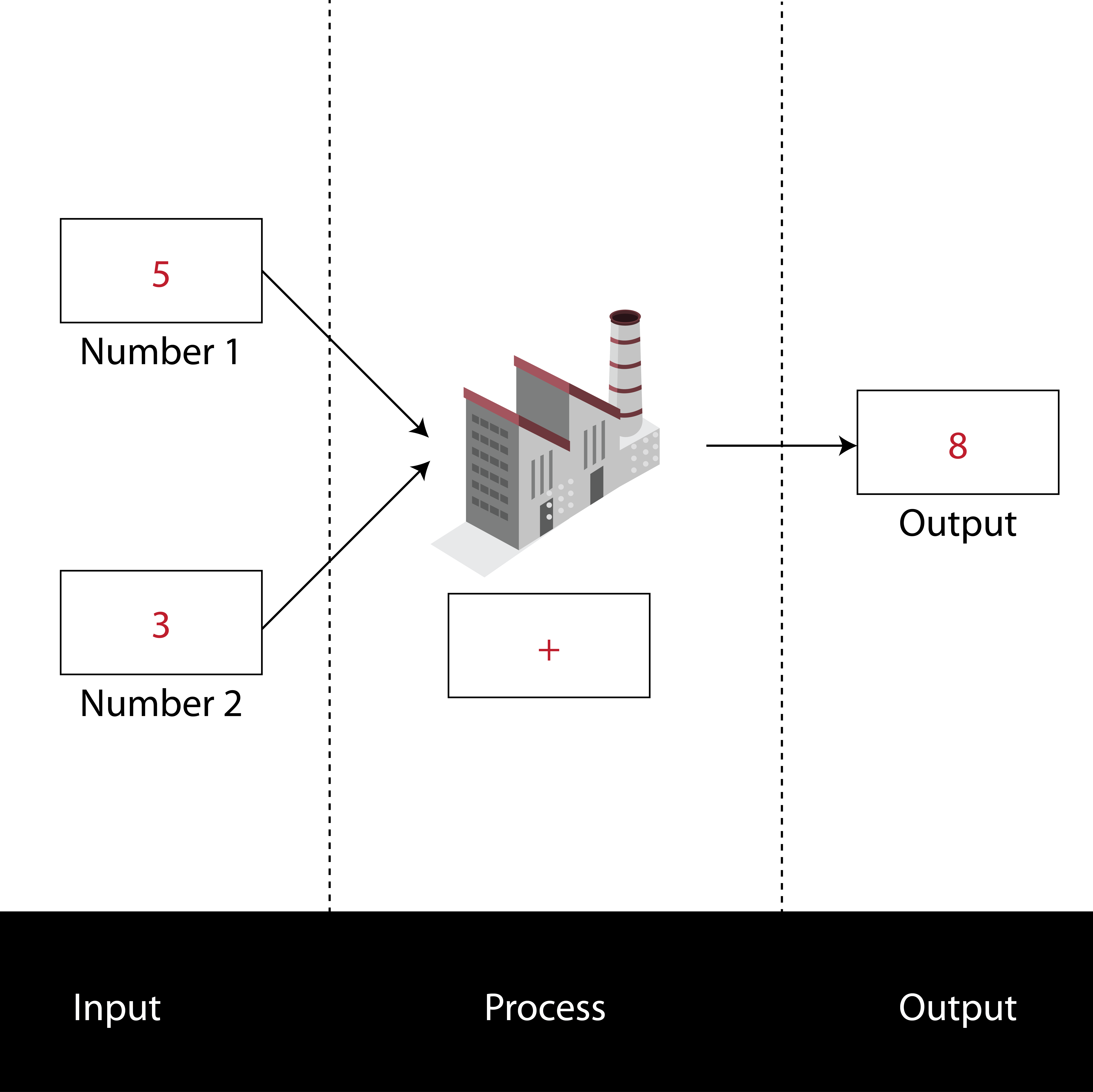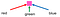# Fundamentals of algorithms

## An algorithm is a sequence of steps that a computer follows. Let’s explore!

Lesson support: https://tinyurl.com/y4pq2jh8

A computer needs a sequence of steps to run through. That sequence is an algorithm and that algorithm can be represented by a computer program.

• None

# Definitions

Abstraction: The process of removing unnecessary detail from a problem.

Algorithm: A process or set of rules to be followed.

Computer program: An implementation of an algorithm (or algorithms).

Decomposition: The act of breaking a problem into a series of sub-problems, with the goal of solving the initial problem.

# Algorithms

To solve a problem you often need to take a step-by-step approach to it. We can call this set of steps an algorithm.

One way of thinking of an algorithm is as something taking an input, applying a process to it to produce the desired output.

Input -> Process -> Output

We can think of an algorithm as a problem-solving machine that performs these three stages.An adding machine that takes two inputs, adds them and outputs the answer. Here 5 + 3 = 8

This fantastic adding machine takes two numbers, adds them and then outputs the result. It is pretty easy to imagine other problem-solving machines that perform other mathematical operations.

To become a useful algorithm, we generally chain these operations together.

Consider how we can calculate a percentage:

The following machine works out that 5/50 is 10%.

Even using the machine diagrams above, things are not always clear. How do you know that 5 must be divided by 50, rather than 50 divided by 5?

An algorithm must be so simple to follow that even a machine can do it. This is not a mistake; computers are simple instruction following machines and if your instructions are not clear enough the machines will not be able to follow them (at least, not well enough to present a correct answer!).

There are a few ways to clearly write the instructions, as shown below:

## Pseudocode

Pseudocode is a way of writing the algorithm without having to agree on which programming language to use. This exposes the algorithm’s nature as a set of instructions.

The adding machine in Pseudocode:

You might notice that there are some extra components here. We needed to tell the adding machine to print out the answer, for example.

# Flowcharts

Flowcharts are a way to show an algorithm, and the flow goes through the instructions to develop the solution to a problem.

The commonly used shapes are shown below:

A classic example of an algorithm represented by a flow chart is “Making a cup of Tea”. The problem with using flowcharts to represent algorithms is the pertinent question: are the actions broken into small enough chunks to be carried out by a machine? One such example is shown below:

# Comparison of pseudocode and flowcharts

Pseudocode and flowcharts are equivalent. Imagine looking at a table of information or a bar graph. Although they look different, they actually show the same information! Pseudocode and flowcharts are the same things in the world of algorithms.

# The Tools to help us solve problems

We have already seen that Pseudocode and flowcharts can help us to describe the algorithms that solve problems. To create the algorithm there are several techniques we can use to help us. One such technique is decomposition.

# Decomposition

How do you eat an Elephant? One tusk at a time.

This “joke” gets to the heart of decomposition. To eat a large animal, split it down into smaller parts that you can eat. To solve a problem, break the problem down into smaller components that you can solve.

You might have heard about things decomposing in biology, which has a similar meaning of organic substances breaking down into their component parts (dead leaves from a tree decompose into their minerals).

Outside of Computer Science decomposition is everywhere. If you want to be a lawyer you need to go to University. To go to University you would need good grades in school. To get good grades in school…I’ll leave it with you to break down that idea more!

Computer Science examples often rely on simple mathematical examples, although they don’t have to be.

1. The fun example:

Break a shopping trip into all of the tasks that are needed to perform this.

2. The simple Maths example

Break down how to calculate a percentage with any two numbers. Explain ALL the steps both in Pseudocode and through a FlowChart.

Why would we want to do this?

Decomposition makes your life much easier. Once you can do the small parts of a puzzle, you find that slotting them together, to get the actual solution is much easier.

To complement decomposition we can use other techniques, one of them being an abstraction.

# Abstraction

However, we can sometimes get confused with the extra details given in a problem. Like a map, we can use the representation of a problem to solve the problem itself without those annoying details. Or we can drive a car and press the accelerator without any real idea of how the car works.

Did you know that each pixel of your computer screen is made up of red, green and blue? Each image is abstracted into simple dots with three colours!An abstraction of a pixel on your computer screen

By removing details from a problem we can identify the problem at hand.

A common example of abstraction is a map. Unnecessary detail is removed from the world until we have a 2-dimensional representation of an area. The complex world of people, landmarks, weather, building, and roads is simplified in such a way that makes it easy to understand what way you need to go.

It turns out that abstraction can help us identify similar problems, so if we solve one problem we know how to solve many similar problems.

An example of this is the study of animals. In animation, you if you know how to draw a dog running you can make a good job of drawing a wolf running — after all, it’s still an animal running.

By reducing a problem to a simpler version through abstraction you enhance your problem-solving skills and techniques.

# Rounding up

Algorithms are extremely important in computing, as we use them to in effect tell the computer what to do.

Algorithms are often used in specific programming languages. Because different people may not understand the same programming language so we can use Pseudocode or Flowcharts to express an algorithm so all readers can understand.

A good way to make problems solvable is to use abstraction or decomposition as tools to help make the algorithm.

Learning Swift